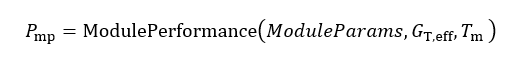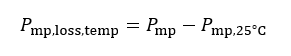# Loss Factor Calculations

Loss factors are computed on a Block by Block basis. A final weighted average is also provided where applicable. The reference points for each of set loss factors quotients are shown in small caps text.### Figure 43. Loss Factor Definitions

The non-STC losses (loss due to lower efficiency at lower irradiance levels, and the loss due to the module temperature coefficient), are detailed as follows:

Reference: Compute the array Pmp at STC conditions (1000 W/m2, 25 °C, air mass 1.5, incidence angle of 90 degrees) and scaled to the effective irradiance. Let this be denoted by Pmp,ηSTC: E.g., @ 200 W/m2, energy output shall be defined as 1/5 the energy at STC. In other words, we assume a linear irradiance dependency of module power.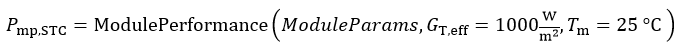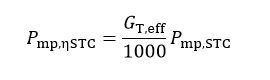Irradiance Loss: Compute the Pmp under actual irradiance conditions but with module temperature fixed at 25 °C. The difference between this Pmp and the previous Pmp will be the energy lost (or gained) due to the module’s response at different radiant intensities.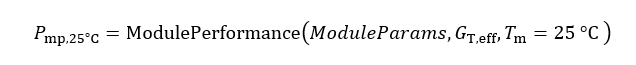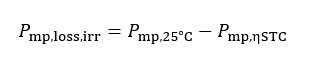# Module Temperature Loss

Temperature loss: Compute the Pmp under real conditions (actual temperature and irradiance). The difference between this Pmp and the previous Pmp will be the energy lost (or gained) due to the modules temperature response; i.e. operation at temperatures other than 25 °C